# 基于数字图像技术对Nano-SiO2改性混凝土力学性能的研究 Study on Mechanical Properties of Nano-SiO2Modified Concrete Based on Digital Image Technology

DOI: 10.12677/NAT.2020.104008, PDF, HTML, XML, 下载: 106  浏览: 201

Abstract: Through digital image processing technology (DIC), the compressive test of nano-modified concrete with different content (0.8%, 1.0%, 1.5%, 2.0%) is carried out, and the CCD low-speed camera is used to monitor the sample loading process in real time, and is based on Match ID. The software analyzes the changing law of the displacement field U under different loads and the changing law of the displacement of different coordinates in the crack development area. Research shows that in the compressive test of nano-modified concrete with different content, the concrete strength is higher when the content is 2.0%. Before the crack appears, the displacement change is small, and the crack develops to the final failure, the displacement change is larger.

1. 引言

2. 原材料及试验设计

2.1. 原材料Table 1. Performance indicators of nanoparticle materialsTable 2. Physical performance indexes of Portland cement

2.2. 试验过程

1、在进行抗压试验前，对混凝土试件进行散斑处理，2、将CCD低速摄像机安装在刚性支架上，调节CCD摄像机位置，使摄像机能对准加载试块；然后固定摄像机位置，避免摄像机移动。3、调节光圈、光源以及对焦，使计算机显示清晰的准备加载试块的图片。4、采用CCD摄像机和Match ID系统采集不同位置校正板信息，保证可以采集到混凝土试块在加载过程中所有的散斑点。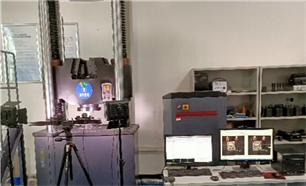(a)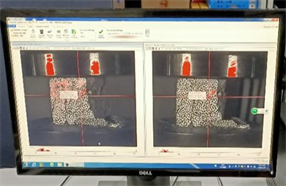(b)

Figure 1. Test equipment. (a) Low-speed camera capture; (b) Acquisition interface

3. 结果与分析

3.1. Match ID位移算法

$\left\{\begin{array}{l}{{x}^{\prime }}_{\xi }={x}_{\xi }+\xi \left({x}_{i},{y}_{j}\right)\\ {{y}^{\prime }}_{j}={y}_{j}+\eta \left({x}_{i},{y}_{j}\right)\end{array}$ (1)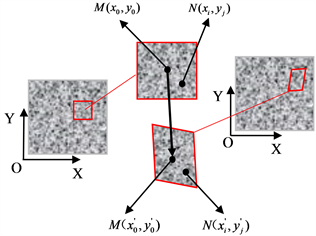Figure 2. Subset before and after deformation

(1)

$\begin{array}{l}{\xi }_{0}\left({x}_{i},{y}_{j}\right)=u\\ {\eta }_{0}\left({x}_{i},{y}_{j}\right)=v\end{array}$ (2)

(2)

$\left\{\begin{array}{l}{{x}^{\prime }}_{i}={x}_{i}+u+\frac{\partial u}{\partial x}\Delta x+\frac{\partial u}{\partial y}\Delta y\\ {{y}^{\prime }}_{j}={y}_{j}+v+\frac{\partial v}{\partial x}\Delta x+\frac{\partial v}{\partial y}\Delta y\end{array}$ (3)

(3)

$\left\{\begin{array}{l}{{x}^{\prime }}_{i}={x}_{i}+u+\frac{\partial u}{\partial x}\Delta x+\frac{\partial u}{\partial y}\Delta y+\frac{1{\partial }^{2}u}{2\partial {x}^{2}}\Delta {x}^{2}+\frac{1{\partial }^{2}u}{2\partial {y}^{2}}\Delta {y}^{2}+{u}_{xy}\Delta x\Delta y\\ {{y}^{\prime }}_{i}={y}_{j}+v+\frac{\partial v}{\partial x}\Delta x+\frac{\partial v}{\partial y}\Delta y+\frac{1{\partial }^{2}v}{2\partial {x}^{2}}\Delta {x}^{2}+\frac{1{\partial }^{2}v}{2\partial {y}^{2}}\Delta {y}^{2}+{v}_{xy}\Delta x\Delta y\end{array}$ (4)

3.2. 试验分析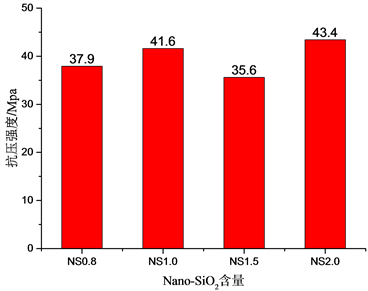Figure 3. Compressive strength of different contents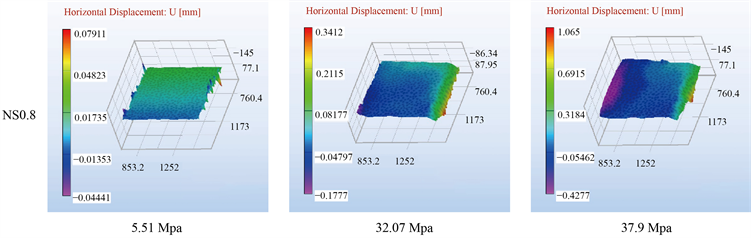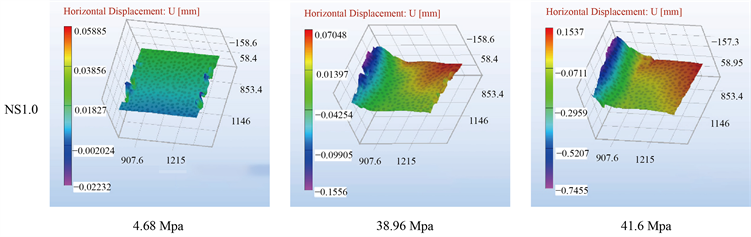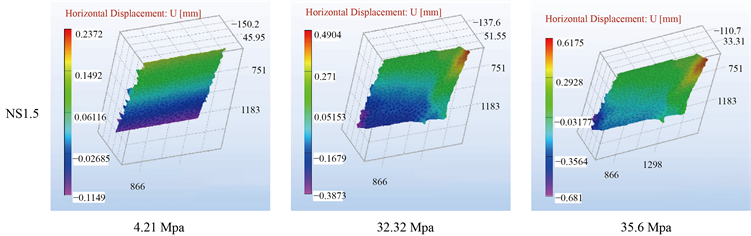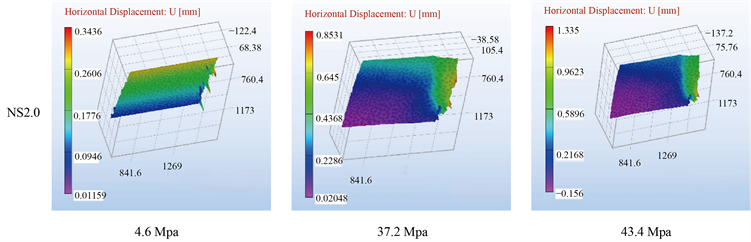Figure 4. Displacement field with different contents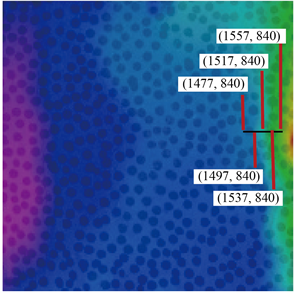Figure 5. NS0.8 point selection analysis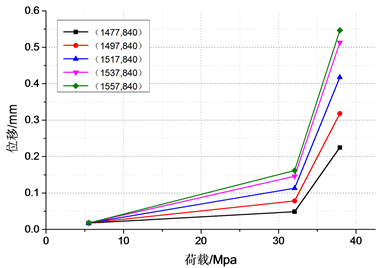Figure 6. NS0.8 coordinate displacement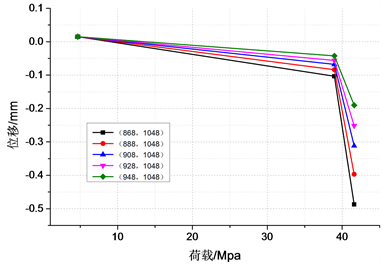Figure 7. NS1.0 coordinate displacement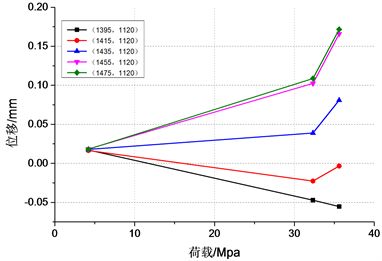Figure 8. NS1.5 coordinate displacement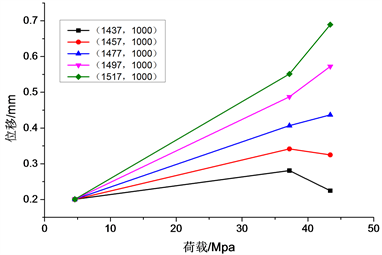Figure 9. NS2.0 coordinate displacement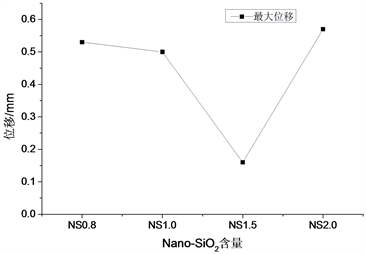Figure 10. Maximum displacement change

4. 结论

(1) 纳米改性混凝土不一定随着纳米材料含量的增加而增大，有可能会影响混凝土的强度，使用纳米改性混凝土应当选取合理的纳米材料含量。Nano-SiO2材料掺量为NS1.5时，较之于NS1.0试样的抗压强度降低幅度约为14.42%，NS2.0强度较大，为43.4 Mpa，较之于NS1.0试样抗压强度增幅约为4.1%。

(2) 加载初期，试样表面位移变化较小，随着荷载的增加，不同含量的纳米改性混凝土(NS0.8、NS1.0、NS1.5、NS2.0)分别在32.09 Mpa、38.96 Mpa、32.32 Mpa、37.2 Mpa出现裂纹，位移变化明显增大，可能只是试样表面出现浅层裂纹，内部尚未开裂，试样NS2.0裂纹开裂早于试样NS1.0。

(3) 基于Match ID软件对裂纹区域选点分析，研究表明：水平方向位移向两边扩展。

(4) 基于数字图像技术，可获取试样裂纹开展全过程水平方向位移和应变数据，根据不同的选点分析区域多个位移变化规律，拓展研究的分析面。

NOTES

*通讯作者。

  叶青. 纳米SiO2与硅粉的火山灰活性的比较[J]. 混凝土, 2001(3): 19-22.  Balapour, M., Joshaghani, A. and Althoey, F. (2018) Nano-SiO2 Contribution to Mechanical, Durability, Fresh and Microstructural Characteristics of Con-crete: A Review. Construction and Building Materials, 181, 27-41. https://doi.org/10.1016/j.conbuildmat.2018.05.266  邢蓉. 纳米SiO2和纳米Fe2O3掺量对混凝土性能的影响[J]. 人民黄河, 2020, 42(1): 131-134+144.  陈坚, 蒋子平. 纳米SiO2对混凝土抗压强度的影响作用[J]. 建筑技术, 2017, 48(1): 38-40.  Morteza, H., Beigi, J.B., et al. (2013) An Experimental Survey on Combined Effects of Fibers and Nanosilica on the Mechanical, Rheological, and Durability Properties of Self-Compacting Concrete. Materials and Design, 50, 1019-1029. https://doi.org/10.1016/j.matdes.2013.03.046  Eskandari, H., Vaghefi, M. and Kowsari, K. (2015) Investigation of Mechanical and Durability Properties of Concrete Influenced by Hybrid Nano Silica and Micro Zeolite. Procedia Materials Science, 11, 594-599. https://doi.org/10.1016/j.mspro.2015.11.084  姚廒, 师阳. 纳米SiO2混凝土力学性能研究[J]. 佳木斯大学学报(自然科学版), 2019, 37(4): 517-519+524.  叶青. 硅溶胶对水泥基材料微观结构和力学性能的影响[J]. 硅酸盐学报, 2008(4): 425-430.  Sutton, M.A., Orteu, J.J. and Schreier, H.W. (2009) Image Correlation for Shape, Motion and Deformation Measurements. Springer, New York.  Hild, F. and Roux, S. (2006) Digital Image Corre-lation: From Displacement Measurement to Identification of Elastic Properties—A Review. Strain, 42, 69-80. https://doi.org/10.1111/j.1475-1305.2006.00258.x  贺体人. 结合数字图像相关技术并采用位移数据开展复合材料本构识别的可行性分析[C]//中国力学学会固体力学专业委员会、国家自然科学基金委员会数理科学部. 2018年全国固体力学学术会议摘要集(上). 2018: 1.  Chu, T.C., Ranson, W.F. and Sutton, M.A. (1985) Appli-cations of Digital-Image-Correlation Techniques to Experimental Mechanics. Experimental Mechanics, 25, 232-244. https://doi.org/10.1007/BF02325092  Pan, B., Qian, K.M., Xie, H.M. and Asundi, A. (2009) Two-Dimensional Digital Image Correlation for In-Plane Displacement and Strain Measurement: A Review. Measure-ment Science and Technology, 20, Article ID: 062001. https://doi.org/10.1088/0957-0233/20/6/062001  李根, 王学志, 张晓飞, 胡柯心, 李春蕊. 基于数字散斑法研究碾压混凝土的有效裂纹扩展量[J]. 混凝土, 2018(9): 9-13.  Ahn, B. and Nutt, S.R. (2010) Strain Map-ping of Al-Mg Alloy with Multiscale Grain Structure Using Digital Image Correlation Method. Experimental Mechanics, 50, 117-123.